Next: Spherical Interfaces Up: Surface Tension Previous: Introduction

# Young-Laplace Equation

Consider an interface separating two immiscible fluids that are in equilibrium with one another. Let these two fluids be denoted 1 and 2. Consider an arbitrary segmentof this interface that is enclosed by some closed curve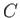. Let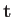denote a unit tangent to the curve, and let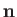denote a unit normal to the interface directed from fluid 1 to fluid 2. (Note thatcirculates aroundin a right-handed manner. See Figure 3.1.) Suppose that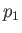andare the pressures of fluids 1 and 2, respectively, on either side of. Finally, let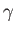be the (uniform) surface tension at the interface.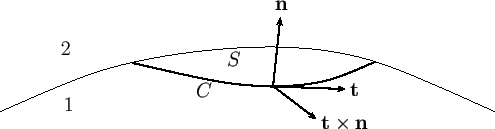The net force acting onis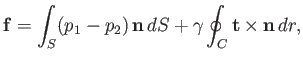(3.1)

where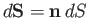is an element of, and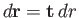an element of. Here, the first term on the right-hand side is the net normal force due to the pressure difference across the interface, whereas the second term is the net surface tension force. Note that body forces play no role in Equation (3.1), because the interface has zero volume. Furthermore, viscous forces can be neglected, because both fluids are static. In equilibrium, the net force acting onmust be zero: that is,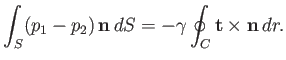(3.2)

(In fact, the net force would be zero even in the absence of equilibrium, because the interface has zero mass.)

Applying the curl theorem (see Section A.22) to the curve, we find that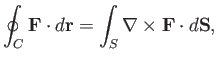(3.3)

whereis a general vector field. This theorem can also be written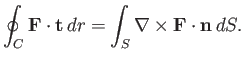(3.4)

Suppose that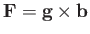, where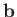is an arbitrary constant vector. We obtain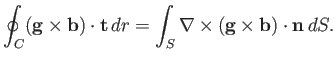(3.5)

However, the vector identity (A.179) yields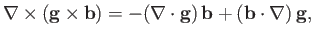(3.6)

asis a constant vector. Hence, we get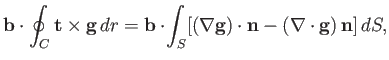(3.7)

where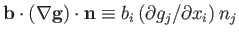. Now, becauseis also an arbitrary vector, the previous equation gives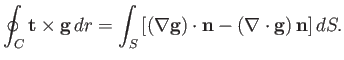(3.8)

Taking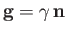, we find that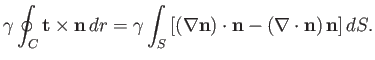(3.9)

But,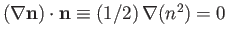, becauseis a unit vector. Thus, we obtain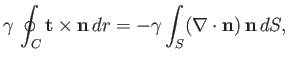(3.10)

which can be combined with Equation (3.2) to give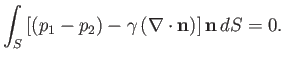(3.11)

Finally, given thatis arbitrary, the previous expression reduces to the pressure balance constraint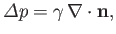(3.12)

where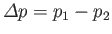. The previous relation is generally known as the Young-Laplace equation, and is named after Thomas Young (1773-1829), who developed the qualitative theory of surface tension in 1805, and Pierre-Simon Laplace (1749-1827) who completed the mathematical description in the following year. The Young-Laplace equation can also be derived by minimizing the free energy of the interface. (See Section 3.8.) Note thatis the jump in pressure seen when crossing the interface in the opposite direction to. Of course, a plane interface is characterized by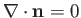. On the other hand, a curved interface generally has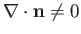. In fact,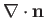measures the local mean curvature of the interface. Thus, according to the Young-Laplace equation, there is a pressure jump across a curved interface between two immiscible fluids, the magnitude of the jump being proportional to the surface tension.Next: Spherical Interfaces Up: Surface Tension Previous: Introduction
Richard Fitzpatrick 2016-03-31